Question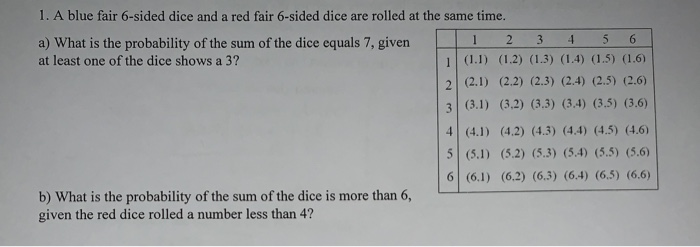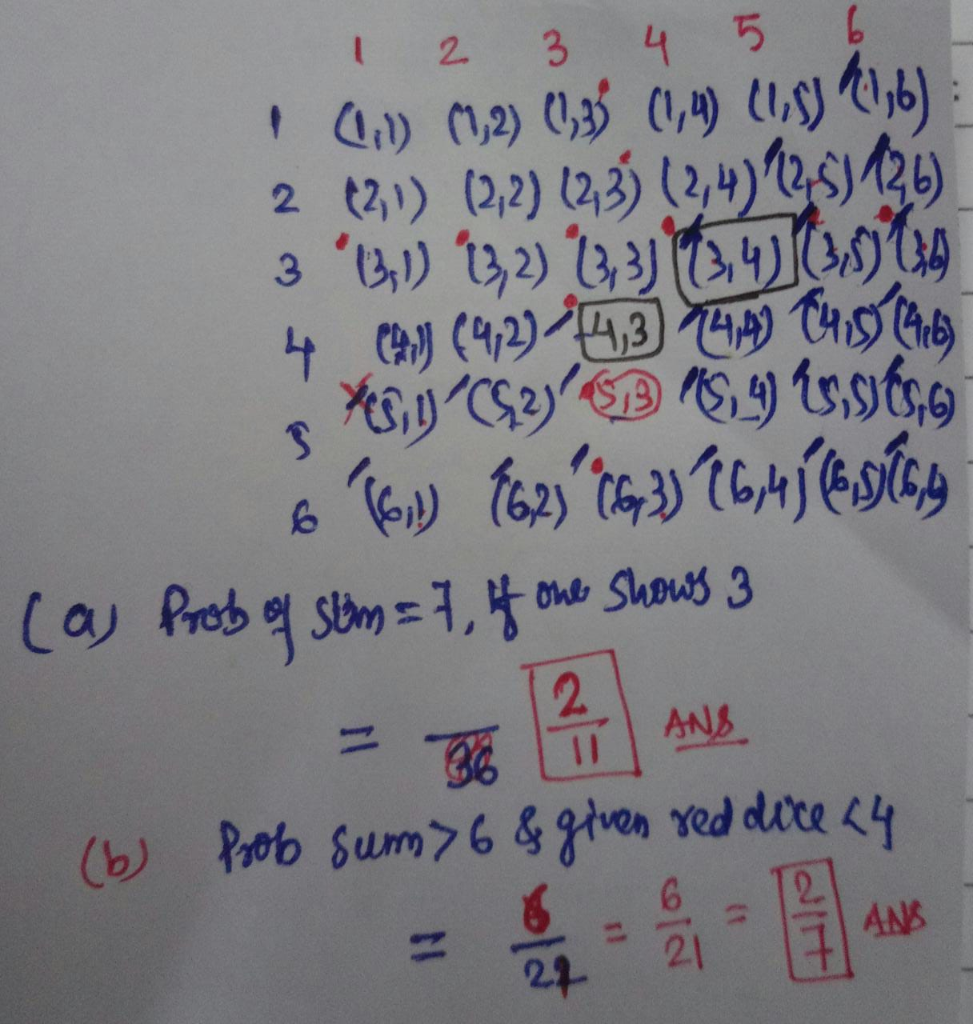#### Earn Coins

Coins can be redeemed for fabulous gifts.

Similar Homework Help Questions
• ### Parametirc test or not: Test statistic: p-value: decision: Is There A Difference Between the Means?

Parametirc test or not:Test statistic:p-value:decision:Is There A Difference Between the Means?6.7 6.2 3.1 310.3 10 5 5.56.9 5.5 3.3 3.110.5 6.3 4.3 5.44.5 4.6 1.8 25.6 5.6 2 2.65.9 6.1 2.1 2.58 11.7 4 4.68 7.4 3.3 3.15.8 5.2 3.1 2.96 7.3 3.0 3.28.7 5.3 2.7 36 5.5 2.1 2.27.2 6.3 3.5 3.25.9 4.6 2.9 3.46 7.4 3 3.37.2 7.8 3.7 3.48.6 9.4 5.1 5.77.2 8.1 2.8 3.15.8 5.4 2.2 1.83.3 4 1.7 1.86.8 5.1 2 1.83.7 3.5 2.2 2.112...

• ### I need help with frequency distribution.. thanks!

In a study of 40 women, the following data of blood potassium levels, in milliequivalents per liter were obtained. Construct a frequency distribution table using 7classes.2.7, 3.2, 3.4, 3.6, 3.6, 3.7, 3.7, 3.8, 3.8, 3.9, 3.9,4.0, 4.1, 4.1, 4.2, 4.2, 4.2, 4.3, 4.3, 4.3, 4.3, 4.4, 4.4, 4.5, 4.5, 4.6, 4.7, 4.7, 4.9, 4.9, 4.9,5.0, 5.1, 5.1, 5.2, 5.3, 5.6, 5.8, 5.8, 6.0

• ### An experiment consists of rolling two fair dice and adding the dots on the two sides...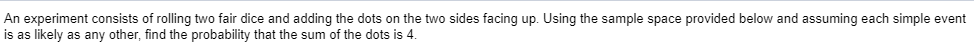An experiment consists of rolling two fair dice and adding the dots on the two sides facing up. Using the sample space provided below and assuming each simple event is as likely as any other, find the probability that the sum of the dots is 4. 1 5 (1,5) First Die (2.5) 1 (1,1) 2 (2.1) 3 (3.1) 4 (4.1) 5 (5.1) 6 (61) 2 (1,2) (2.2) (3,2) (4,2) (5,2) (6,2) Second Die 3 4 (1,3) (1.4) (2,3) (2.4) (3,3)...

• ### Three fair six-sided dice are rolled. a) What is the probability of seeing {1, 3, 6}?...

Three fair six-sided dice are rolled. a) What is the probability of seeing {1, 3, 6}? b) What is the probability of seeing {1, 4, 4}? c) What is the probability of seeing {2, 2, 2} ? d) What is the probability of seeing at least one 6? e) What is the probability that the sum of all three dice is 16? f) What is the probability of seeing exactly two even numbers?

• ### Two fair (6-sided) dice are rolled. If you are told that the two dice end up...

Two fair (6-sided) dice are rolled. If you are told that the two dice end up with two different face values, what is the probability that one die has a face value of 6?

• ### 1. Suppose 7 dice are rolled. The dice are 6-sided and fair. a). Find the probability...

1. Suppose 7 dice are rolled. The dice are 6-sided and fair. a). Find the probability that more than 5 dice show 2 or less (you may leave your answer in unsimplified form). I found this answer to be 5/729= 0.006859 b). Suppose we roll 7 dice and count the number showing 2 or less. We repeat this experiment over and over, each time counting the number showing 2 or less. What should we expect to compute as an average...

• ### n the table below, earthquake magnitudes are given for any region for the last 100 years....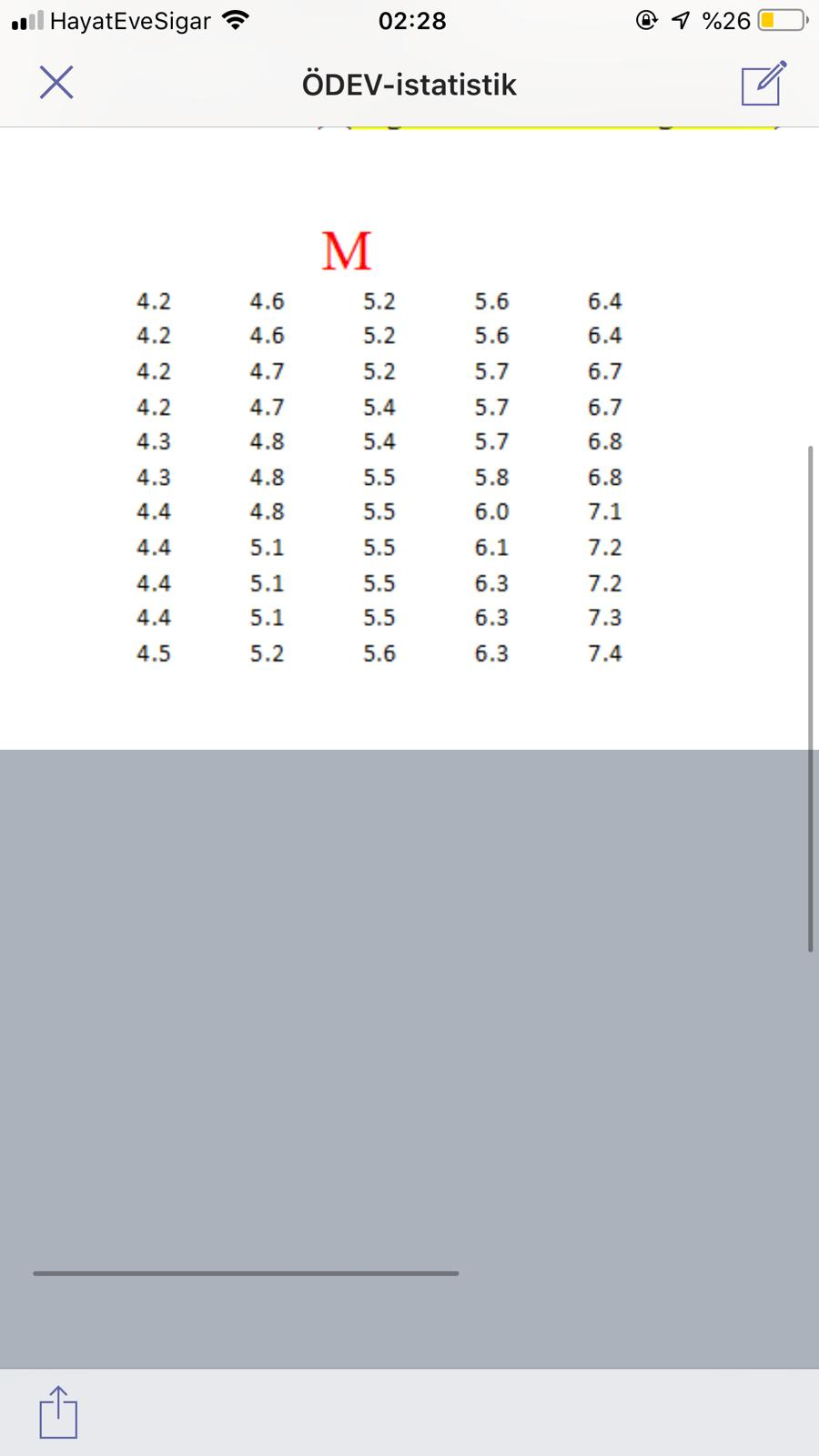n the table below, earthquake magnitudes are given for any region for the last 100 years. Convert each value in this table to your own values ​​to get a new table. your new values A) frequency table and create histogram find B)standard deviation, find values C) ,%variation coefficients find values D) mode and median find values use the formula below for conversion Mnew=M-log10(N) N=33 please show which item you solved when solving Sample (A B C D) single digit after...

• ### 4. Three fair dice have different colors: red, blue, and yellow. These three dice are rolled...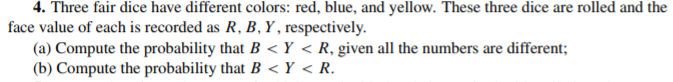4. Three fair dice have different colors: red, blue, and yellow. These three dice are rolled and the face value of each is recorded as R, B, Y, respectively. (a) Compute the probability that B< Y < R, given all the numbers are different; (b) Compute the probability that B< Y< R.

• ### Two fair six-sided dice are rolled. What is the probability that one die shows exactly three...

Two fair six-sided dice are rolled. What is the probability that one die shows exactly three more than the other die (for example, rolling a 1 and 4, or rolling a 6 and a 3)

• ### You roll a pair of fair 6-sided dice: a red die and a blue die. (a)...

You roll a pair of fair 6-sided dice: a red die and a blue die. (a) Consider event A: {the outcome of the red die is more than 3} and event B: {the outcome of the red die is less than 5}. Given that event A occurs, what is the probability that event B occurs? (b) Are A and B mutually exclusive (i.e., disjoint)? (c) Are A and B independent? (d) Calculate the probability of event C: {the outcome of...